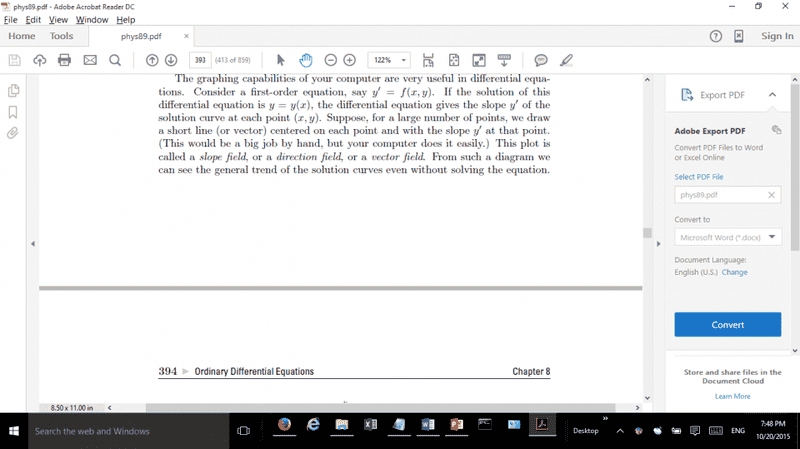# Functions of more than one variable nomenclature

## Homework Statementn/a

## The Attempt at a Solution

##y'=f(x.y)## is a function of two variables. ##y=y(x)## is a function of only one variable. How can they be related? Clearly ##y(x) = f(x) \neq f(x,y)##
Thanks

## Answers and Replies

Perhaps ##f(x) \neq y(x)##

Mark44
Mentor

## Homework Statement

View attachment 90522

n/a

## The Attempt at a Solution

##y'=f(x.y)## is a function of two variables. ##y=y(x)## is a function of only one variable. How can they be related? Clearly ##y(x) = f(x) \neq f(x,y)##
Thanks
The right side of the differential equation y' = f(x, y) involves expressions in both x and y. For example, something like y' = 2x + 3y. Here f is a function that maps a pair of numbers (x, y) to 2x + 3y.

We generally assume that y is related to x in some way.
Perhaps ##f(x) \neq y(x)##
Correct.